# Civil Engineering - UPSC Civil Service Exam Questions

41.

A train starts from rest on a curved track of radius 800 m. Its speed increases uniformly and after 3 minutes it is 72 km/hr. Its normal acceleration after 2 minutes is

 A.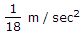B.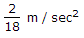C.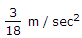D.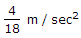Explanation:

No answer description available for this question. Let us discuss.

42.

A hollow cylinder made of wood (sp. gr. = 0.8) has an external diameter of 1.0 m and an interal diameter of 0.6 m. It floats in water with its axis vertical and is in stable equilibrium. This is possible only when the length of the cylinder is equal to or less than

 A. 0.72 m B. 0.95 m C. 1.03 m D. 1.20 m

Explanation:

No answer description available for this question. Let us discuss.

43.

In a fillet weld the weakest section is the

 A. smaller side of the fillet B. throat of the fillet C. side perpendicular to force D. side parallel to force

Explanation:

No answer description available for this question. Let us discuss.

44.

A beam ABC is simply-supported at A and B with an overhang BC as shown in the given figure. It carries loads as shown in the figure. If both the reactions are equal then W will be equal to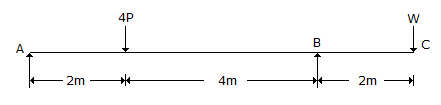A. 8P/5 B. 5P/8 C. 5P/4 D. 4P/5

Explanation:

No answer description available for this question. Let us discuss.

45.

Which one of the following is the correct statement ?
Smaller size of aggregates in a concrete mix :

 A. provides larger surface area for bonding with the mortar matrix which increases the compressive strength and reduces the stress concentration at the mortar-aggregate interface B. is economical as the concrete mix is more dense C. requires lesser cement for a particular water-cement ratio and hence is economical D. is beneficial as these aggregates can pass through the reinforcement bars more easily